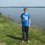# Proving a combinatorial identity

Prove that

${n \choose 0}^2 + {n \choose 1}^2 + {n \choose 2}^2 + \dots + {n \choose n}^2 = {2n \choose n}.$

Like with most such problems, there are probably many ways to prove this. I proved this by showing that the LHS is an alternative way of choosing $n$ objects from a group of $2n$ distinct objects. Divide this group in two groups $A,B$ each with size $n$. You can choose any number of objects from group $A$, call this number $k$. This can be done in $n \choose k$ ways. Obviously, $0 \leq k \leq n$. Because you pick $n$ objects in total, you still have to choose $n-k$ objects from group $B$, which can be done in ${n \choose {n-k}}$ ways. But we know that for every $0 \leq k \leq n$ with nonnegative $n,k$, that

${n \choose {n-k}} = {n \choose k},$

so choosing $n-k$ objects from group $B$ can also be done in $n \choose k$ ways. Therefore,

$\sum\limits_{k=0}^n {n \choose k}^2 = {2n \choose n},$

which is what we wanted.

Like I said, there are probably other ways to prove this identity, e.g. by using the binomial theorem. Does anyone have an idea?Note by Tim Vermeulen
7 years ago

This discussion board is a place to discuss our Daily Challenges and the math and science related to those challenges. Explanations are more than just a solution — they should explain the steps and thinking strategies that you used to obtain the solution. Comments should further the discussion of math and science.

When posting on Brilliant:

• Use the emojis to react to an explanation, whether you're congratulating a job well done , or just really confused .
• Ask specific questions about the challenge or the steps in somebody's explanation. Well-posed questions can add a lot to the discussion, but posting "I don't understand!" doesn't help anyone.
• Try to contribute something new to the discussion, whether it is an extension, generalization or other idea related to the challenge.

MarkdownAppears as
*italics* or _italics_ italics
**bold** or __bold__ bold
- bulleted- list
• bulleted
• list
1. numbered2. list
1. numbered
2. list
Note: you must add a full line of space before and after lists for them to show up correctly
paragraph 1paragraph 2

paragraph 1

paragraph 2

[example link](https://brilliant.org)example link
> This is a quote
This is a quote
    # I indented these lines
# 4 spaces, and now they show
# up as a code block.

print "hello world"
# I indented these lines
# 4 spaces, and now they show
# up as a code block.

print "hello world"
MathAppears as
Remember to wrap math in $$ ... $$ or $ ... $ to ensure proper formatting.
2 \times 3 $2 \times 3$
2^{34} $2^{34}$
a_{i-1} $a_{i-1}$
\frac{2}{3} $\frac{2}{3}$
\sqrt{2} $\sqrt{2}$
\sum_{i=1}^3 $\sum_{i=1}^3$
\sin \theta $\sin \theta$
\boxed{123} $\boxed{123}$

Sort by:

I have a method, $\normalsize(1+x)^{n}(1+\frac{1}{x})^{n} = [{n \choose 0}+{n \choose 1}x +{n \choose 3}x^{2}....+{n \choose n}x^{n}] \times [{n \choose 0}+{n \choose 1}\frac{1}{x} + {n \choose 2}\frac{1}{x^{2}}....+{n \choose n}\frac{1}{x^{n}}]$ So,terms on R.H.S which are independent of $x$ are $\normalsize{n \choose 0}^{2} + {n \choose 1}^{2} +...........+ {n \choose n}^{2}.$ L.H.S= $\normalsize\frac{(1+x)^{2n}}{x^{n}}$ So term independent of $x$ on L.H.S = $\normalsize{2n \choose n}.$ Hence proved.Please correct me If I am wrong....!

- 7 years ago

How do you mean "L.H.S= $\normalsize\frac{(1+x)^{2n}}{x^{n}}$"?

- 7 years ago

$\large(1+x)^{n}(1+\frac{1}{x})^{n}= (1+x)^{n}(\frac{1+x}{x})^{n}= (1+x)^{n}\frac{(1+x)^{n}}{x^{n}}=\frac{(1+x)^{2n}}{x^{n}}.$ Now for being independent of $x$ in L.H.S, the power of $x$ in the expansion of numerator must be $n$, so to be canceled out by the denominator one.So coefficient of $\large x^{n}= {2n \choose n}$.Please correct me..

- 7 years ago

That's really cool. I've seen many combinatorial proofs in which they prove that the coefficients of say $x$ are equal, or $x^n$, but never that the coefficients of $x^0$ are equal, i.e. the constant term. Well done!

- 7 years ago

This is just a special case of the Vandermonde identity with m=r=n. Some proofs can be found on that wikipedia page. You can also prove Vandermonde simply by induction on m.

- 7 years ago

Another approach would be to compare the coefficients of $(x+1)^{2n}$ and $(x+1)^n(x+1)^n$. The coefficient of $x^a$ ($1 \leq a \leq n$) in the $L.H.S$ is ${2n \choose a}$, and that in the $R.H.S$ is the sum of all possible values of ${n \choose i}{n \choose j}$, where $i$ and $j$ are non-negative integers which sum to $a$ (this is because in $(x+1)^n(x+1)^n$, we can take any degree of $x$ from the first bracket, which is $i$, and the other degree of $x$ from the second bracket, which is $j$, and for their product to be equal to $a$, we must have $i+j= a$ ). Comparing coefficients and setting $a= n$, the result follows.

Edit:- After revising my proof, I found out that it is somewhat analogous to that submitted by Tim V. In the proof in the discussions body, a group of $2n$ objects is divided into $2$ groups of $n$ objects, and $i$ objects are chosen from the first group, $n-i$ from the other. Basically in my proof, a collection of $2n$ $x$s ($(x+1)^{2n}$) is divided into two groups consisting of $x$s (the two brackets in the $R.H.S$). From the first bracket, $i$ elements are chosen, and $n-i$ are chosen from the second one. So I don't think my proof is different from that already posted. :(

That's not a problem, it's just another way of explaining it :)

- 7 years ago

Hi, It's called "convolution of generating functions", it can be used to derive many more such identities!

- 7 years ago